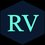# HELP NEEDED !!

Can anyone please help me in solving this $\displaystyle{\sum _{ i=0 }^{ 20 }{ \sum _{ j=i+1 }^{ 20 }{ { { \left( \begin{matrix} 20 \\ i \end{matrix} \right) } } } } \left( \begin{matrix} 20 \\ j \end{matrix} \right) }$

Its answer is $\frac { { 2 }^{ 40 }-\left( \begin{matrix} 40 \\ 20 \end{matrix} \right) }{ 2 }$Note by Vighnesh Raut
6 years, 2 months ago

This discussion board is a place to discuss our Daily Challenges and the math and science related to those challenges. Explanations are more than just a solution — they should explain the steps and thinking strategies that you used to obtain the solution. Comments should further the discussion of math and science.

When posting on Brilliant:

• Use the emojis to react to an explanation, whether you're congratulating a job well done , or just really confused .
• Ask specific questions about the challenge or the steps in somebody's explanation. Well-posed questions can add a lot to the discussion, but posting "I don't understand!" doesn't help anyone.
• Try to contribute something new to the discussion, whether it is an extension, generalization or other idea related to the challenge.
• Stay on topic — we're all here to learn more about math and science, not to hear about your favorite get-rich-quick scheme or current world events.

MarkdownAppears as
*italics* or _italics_ italics
**bold** or __bold__ bold
- bulleted- list
• bulleted
• list
1. numbered2. list
1. numbered
2. list
Note: you must add a full line of space before and after lists for them to show up correctly
paragraph 1paragraph 2

paragraph 1

paragraph 2

[example link](https://brilliant.org)example link
> This is a quote
This is a quote
    # I indented these lines
# 4 spaces, and now they show
# up as a code block.

print "hello world"
# I indented these lines
# 4 spaces, and now they show
# up as a code block.

print "hello world"
MathAppears as
Remember to wrap math in $$ ... $$ or $ ... $ to ensure proper formatting.
2 \times 3 $2 \times 3$
2^{34} $2^{34}$
a_{i-1} $a_{i-1}$
\frac{2}{3} $\frac{2}{3}$
\sqrt{2} $\sqrt{2}$
\sum_{i=1}^3 $\sum_{i=1}^3$
\sin \theta $\sin \theta$
\boxed{123} $\boxed{123}$

## Comments

Sort by:

Top Newest

$\displaystyle{\sum _{ i=0 }^{ 20 }{ \sum _{ j=i+1 }^{ 20 }{ { { \left( \begin{matrix} 20 \\ i \end{matrix} \right) } } } } \left( \begin{matrix} 20 \\ j \end{matrix} \right) }$ $= \displaystyle \sum_{i=0}^{20} \binom{20}{i} \times \left[ \binom{20}{i+1}+\binom{20}{i+2}+.....+\binom{20}{20}\right]$ $=\text{ Sum of the product of every possible combinations of two out of} \binom{20}{0} , \binom{20}{1}, ...., \binom{20}{20}=\lambda (say)$

$\left[ \binom{20}{0} +\binom{20}{1}+ ....+ \binom{20}{20}\right]^2= \binom{20}{0}^2+ \binom{20}{1}^2+ ....+ \binom{20}{20}^2 +2 \lambda$

$2^{40}=\binom{40}{20}+2\lambda$

$\implies \lambda=\dfrac{2^{40}-\binom{40}{20}}{2}$

Note :

$\displaystyle \sum_{k=0}^n \binom{n}{k}^2=\binom{2n}{n}$

- 6 years, 2 months ago

Log in to reply

Thank you so much sir.... It is a very detailed solution...Understood the process..Once again thanks..

- 6 years, 2 months ago

Log in to reply

Thanks Sir for the detailed solution.

- 6 years, 2 months ago

Log in to reply

From where did you get this question?

- 6 years, 2 months ago

Log in to reply

It came in my mock mains test..

- 6 years, 2 months ago

Log in to reply

Ok,which coaching centre?

- 6 years, 2 months ago

Log in to reply

Career Launcher..

- 6 years, 2 months ago

Log in to reply

ok thanx!

- 6 years, 2 months ago

Log in to reply

where have you joined??

- 6 years, 2 months ago

Log in to reply

I am 14 right now(going to be 15 on may 12),I don't go to any coaching centre.Sorry.

- 6 years, 2 months ago

Log in to reply

oh ok....

- 6 years, 2 months ago

Log in to reply

×

Problem Loading...

Note Loading...

Set Loading...